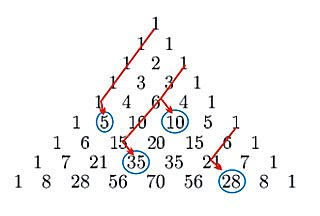18.440 | Spring 2014 | Undergraduate
Probability and Random Variables
Course Description
This course introduces students to probability and random variables. Topics include distribution functions, binomial, geometric, hypergeometric, and Poisson distributions. The other topics covered are uniform, exponential, normal, gamma and beta distributions; conditional probability; Bayes theorem; joint …
Course Info
Instructor
Departments
Topics
Learning Resource Types
assignment Problem Sets